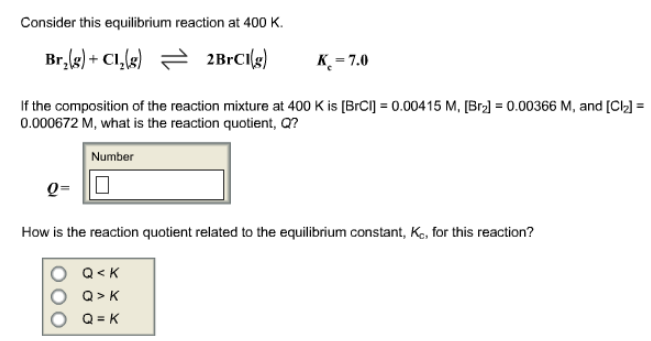# Problem: Consider this equilibrium reaction at 400 K. Br2 (g) + Cl2 (g) ⇌ 2BrCl (g)        Kc = 7.0 If the composition of the reaction mixture at 400 K is [BrCl] = 0.00415 M, [Br2] = 0.00366 M, and [Cl2] = 0.000672 M, what is the reaction quotient, Q? How is the reaction quotient related to the equilibrium constant, Kc, for this reaction?            (i) Q &lt; K            (ii) Q &gt; K           (iii) Q = K

###### FREE Expert Solution
87% (354 ratings)###### Problem Details

Consider this equilibrium reaction at 400 K.

Br2 (g) + Cl2 (g) ⇌ 2BrCl (g)        Kc = 7.0

If the composition of the reaction mixture at 400 K is [BrCl] = 0.00415 M, [Br2] = 0.00366 M, and [Cl2] = 0.000672 M, what is the reaction quotient, Q?

How is the reaction quotient related to the equilibrium constant, Kc, for this reaction?

(i) Q < K

(ii) Q > K

(iii) Q = K